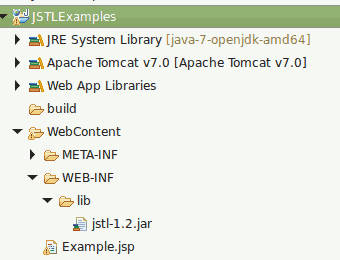# JSTL Check Equals(==), not equals(!=)

JSTL Check Equals(==), not equals(!=) explains about how to use logical conditions(equals, not equals etc) with JSTL tag

Consider a JSP Page where you need to check a value whether it is equals or not equals and process accordingly, in that scenario,  you can follow this example.

On the following table, I am showing 2 different ways (Method 1 & Method 2), you can achieve equals & not equals in JSTL

Logical operation JSTL Method 1 JSTL Method 2
equals eq ==
not equals ne !=
###### Required Libraries

Following jar must be in classpath

1. jstl-1.2.jar

## Project Structure## JSTL Check Equals(eq), not equals(ne) (Method 1)

```<%@ taglib uri="http://java.sun.com/jsp/jstl/core" prefix="c"%>
<%@ taglib uri="http://java.sun.com/jsp/jstl/functions" prefix="fn"%>
<html>
<title>Check equals(eq), not equals(ne)</title>
<body>

<%
// you can also set the values into request scope same as using c:set
request.setAttribute("count", 5);
%>
<!-- you can check "equals(eq)" two ways -->
<c:if test="\${count eq 5}">
count equals to 5<br />
</c:if>

<!-- you can check "not equals(ne)" two ways -->
<c:if test="\${count ne 4}">
count is not equals 4<br />
</c:if>
</body>
</html>
```
###### Output
```count equals to 5
count is not equals 4
```

## JSTL Check Equals(==), not equals(!=) (Method 2)

```<%@ taglib uri="http://java.sun.com/jsp/jstl/core" prefix="c"%>
<%@ taglib uri="http://java.sun.com/jsp/jstl/functions" prefix="fn"%>
<html>
<title>Check equals(==), not equals(!=)</title>
<body>

<%
// you can also set the values into request scope same as using c:set
request.setAttribute("count", 5);
%>
<!-- you can check "equals(==)" two ways -->
<c:if test="\${count == 5}">
count equals to 5<br />
</c:if>

<!-- you can check "not equals(!=)" two ways -->
<c:if test="\${count != 4}">
count is not equals 4<br />
</c:if>
</body>
</html>
```
###### Output
```count equals to 5
count is not equals 4
```

2 Responses to "JSTL Check Equals(==), not equals(!=)"
1. Ridhuvarshan 2013-06-28 13:44:19.0